## A small planet having a radius of 1000 km exerts a gravitational force of 100 N on an object that is 500 km above its surface. If this objec

Question

A small planet having a radius of 1000 km exerts a gravitational force of 100 N on an object that is 500 km above its surface. If this object is moved 500 km farther from the planet, the gravitational force on it will be

in progress 0
6 months 2021-07-19T08:46:52+00:00 1 Answers 68 views 0

56.25 N

Explanation:

Given,

Radius of the planet, r = 1000 km

Gravitational force, F = 100 N

Initial distance from the planet, d = 500 km

Final distance from the planet, D = 1000 km

Lets assume, mass of planet = M

mass of the object = m.

As per the gravitational law, the force due to gravity is given as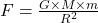where, G = gravitational constant

R =  distance between the two objects.

Case 1: when the object is at a distance of 500 km from the planet,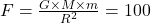Here, R = 1000 + 500 = 1500 km, so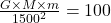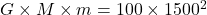Case 2: when the object is at a distance of 1000 km from the planet, so

now, R = 1000 + 1000 = 2000 km

Now the gravitational force, F’ will be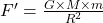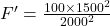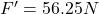Thus, the gravitational force will now be reduced to 56.25 N.